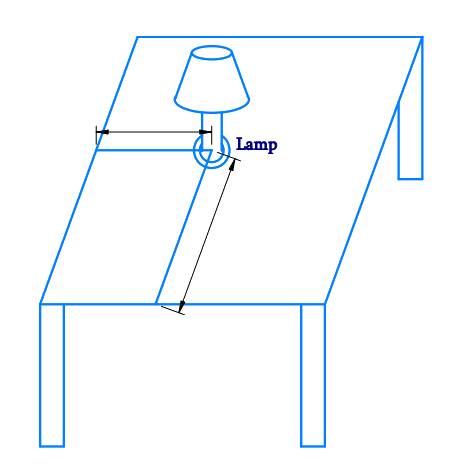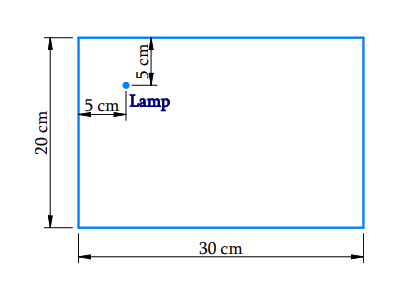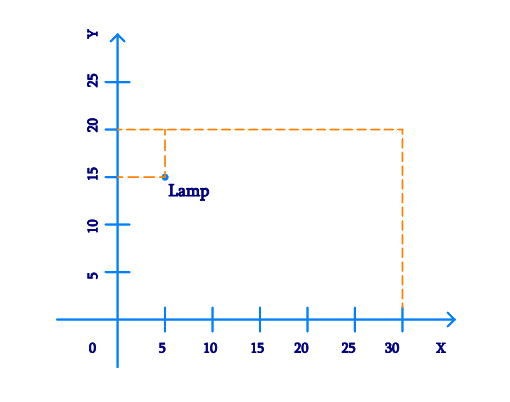In the verge of coronavirus pandemic, we are providing FREE access to our entire Online Curriculum to ensure Learning Doesn't STOP!

# Ex.3.1 Q1 Coordinate Geometry Solution - NCERT Maths Class 9

Go back to  'Ex.3.1'

## Question

How will you describe the position of a table lamp on your study table to another person?

Video Solution
Coordinate Geometry
Ex 3.1 | Question 1

## Text Solution

Steps:The above figure is a $$\rm3-D$$ shows a study table, on which a study lamp is placed.

Now let us consider only the top surface of the table which becomes a $$\rm2-D$$ rectangle figure.From the Figure Above,

• Consider the lamp on the table as a point
• Consider the table as a $$\rm2-D$$ plane.

The table has a shorter side ($$20\,\rm cm$$) and a longer side ($$30\,\rm cm$$).

• Let us measure the distance of the lamp from the shorter side and the longer side.
• Let us assume
• Distance of the lamp from the shorter side is $$5 \rm\;{cm}.$$
• Distance of the lamp from the longer side is $$5 \rm\;{cm}.$$Therefore, we can conclude that the position of the lamp on the table can be described with respect to the order of the axes as ($$5,15$$).

Video Solution
Coordinate Geometry
Ex 3.1 | Question 1

Learn from the best math teachers and top your exams

• Live one on one classroom and doubt clearing
• Practice worksheets in and after class for conceptual clarity
• Personalized curriculum to keep up with school